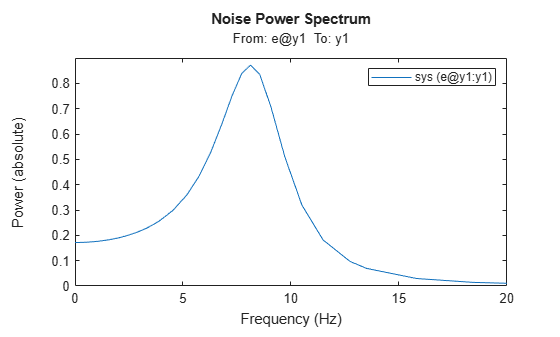# spectrumplot

Plot disturbance spectrum of linear identified models

## Syntax

```spectrumplot(sys) spectrumplot(sys,line_spec) spectrumplot(sys1,line_spec1,...,sysN,line_specN) spectrumplot(ax, ___) spectrumplot(___,plot_options) spectrumplot(sys,w) h = spectrumplot(___) ```

## Description

`spectrumplot(sys)` plots the disturbance spectrum of the model, `sys`. The software chooses the number of points on the plot and the plot frequency range.

If `sys` is a time-series model, its disturbance spectrum is the same as the model output spectrum. You generally use this function with time-series models.

`spectrumplot(sys,line_spec)` uses `line_spec` to specify the line type, marker symbol, and color.

`spectrumplot(sys1,line_spec1,...,sysN,line_specN)` plots the disturbance spectrum for one or more models on the same axes.

You can mix `sys`,`line_spec` pairs with `sys` models as in `spectrumplot(sys1,sys2,line_spec2,sys3)`. `spectrumplot` automatically chooses colors and line styles in the order specified by the `ColorOrder` and `LineStyleOrder` properties of the current axes.

`spectrumplot(ax, ___)` plots into the axes with handle `ax`. All input arguments described for the previous syntaxes also apply here.

`spectrumplot(___,plot_options)` uses `plot_options` to specify options such as plot title, frequency units, etc. All input arguments described for the previous syntaxes also apply here.

`spectrumplot(sys,w)` uses `w` to specify the plot frequencies.

• If `w` is specified as a 2-element cell array, `{wmin, wmax}`, the plot spans the frequency range `{wmin, wmax}`.

• If `w` is specified as vector, the spectrum is plotted for the specified frequencies.

Specify `w` as `radians/time_unit`, where `time_unit` must equal `sys.TimeUnit`.

`h = spectrumplot(___)` returns the handle to the spectrum plot. You use the handle to customize the plot. All input arguments described for the previous syntaxes also apply here.

## Input Arguments

 `sys` Identified linear model. `line_spec` Line style, marker, and color of both the line and marker, specified as a character vector. For example, `'b'`, `'b+:'`. For more information, see Line Properties . `ax` Plot axes handle. Specify as a double-precision value. You can obtain the current axes handle by using the function, `gca`. `plot_options` Plot customization options. Specify as a plot options object. You use the command, `spectrumoptions`, to create `plot_options`. For more information, type `help spectrumoptions`. `w` Frequency range. Specify in `radians/time_unit`, where `time_unit` must equal `sys.TimeUnit`.

## Output Arguments

 `h` Plot handle for spectrum plot, returned as a double-precision value.

## Examples

collapse all

Obtain the identified model.

```load iddata9 z9 sys = ar(z9,4);```

Plot the output spectrum for the model.

`spectrumplot(sys);`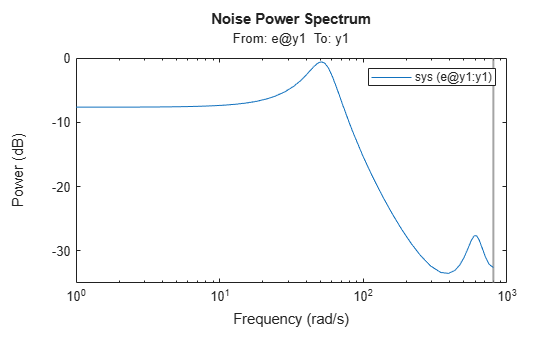Obtain the identified model.

```load iddata9 z9 sys = ar(z9,4);```

Specify the line width and marker style for the spectrum plot.

`spectrumplot(sys,'k*--');`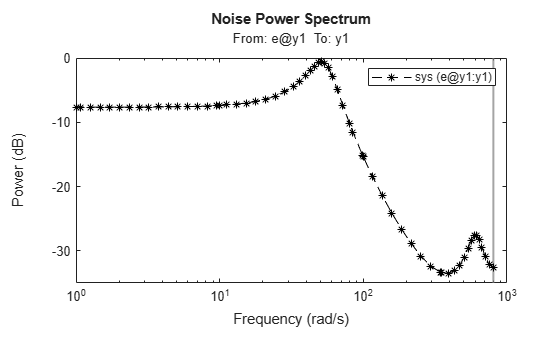`'k*--'`, specifies a dashed line (`--`) that is black (`k`), with star markers (`*`).

Obtain multiple identified models.

```load iddata9 z9 sys1 = ar(z9,4); sys2 = ar(z9,2);```

Plot the output spectrum for both models.

```spectrumplot(sys1,'b*-',sys2,'g^:'); legend('sys1','sys2');```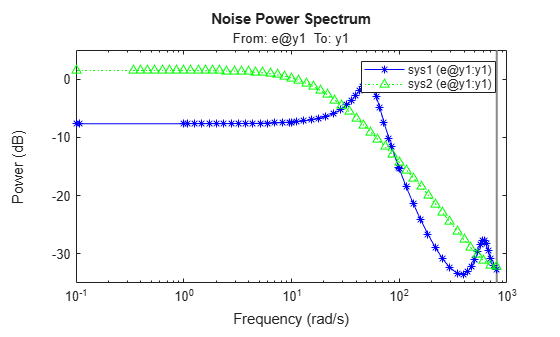Obtain the axes handle for a plot.

```load iddata9 z9 sys1 = ar(z9,4); spectrumplot(sys1);```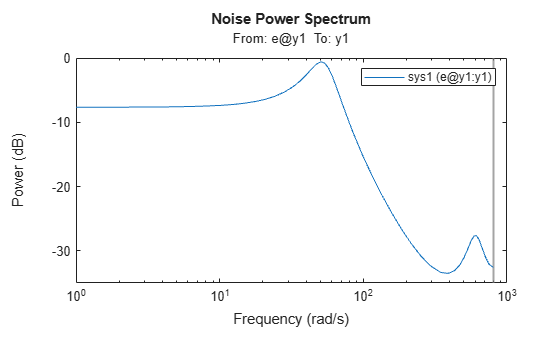`ax = gca;`

`ax` is the handle for the spectrum plot axes.

Plot the output spectrum for another model on the specified axes.

```sys2 = ar(z9,2); hold on; spectrumplot(ax,sys2,'r*--'); legend('sys1','sys2');```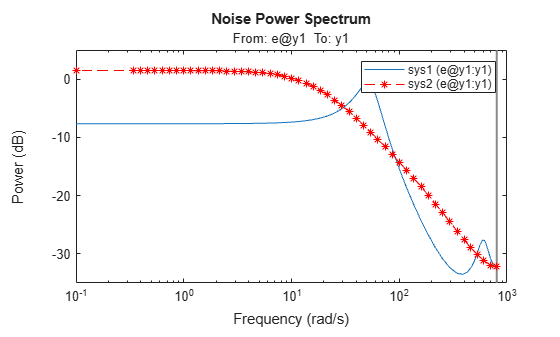Specify the plot options.

```plot_options = spectrumoptions; plot_options.FreqUnits = 'Hz'; plot_options.FreqScale = 'linear'; plot_options.Xlim = {[0 20]}; plot_options.MagUnits = 'abs';```

Estimate an `AR` model.

```load iddata9 z9 sys = ar(z9,4);```

Plot the output spectrum for the model.

`spectrumplot(sys,plot_options);`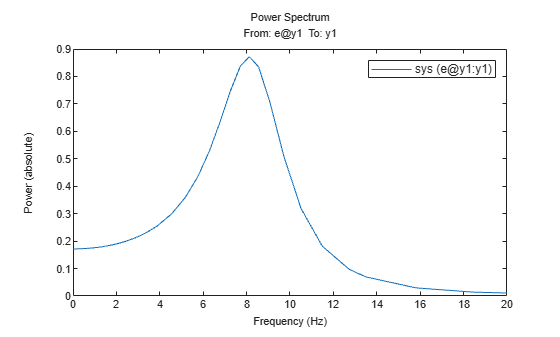Obtain the identified model.

```load iddata9 z9 sys = ar(z9,4);```

Specify the frequency range for the output spectrum plot for the model.

`spectrumplot(sys,{1,1000});`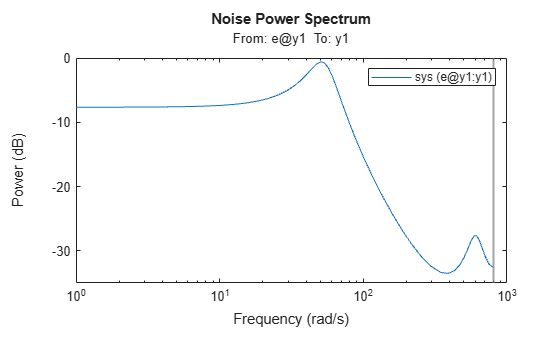The 2-element cell array `{1,1000}` specifies the frequency range from 1 rad/s to 1000 rad/s.

Obtain the identified model.

```load iddata9 z9 sys = ar(z9,4);```

Get the plot handle for the model spectrum plot.

`h = spectrumplot(sys);`(Optional) Specify the plot options, using the plot handle.

`setoptions(h,'FreqUnits','Hz','FreqScale','linear','Xlim',{[0 20]},'MagUnits','abs');`